# Texas Go Math Grade 5 Lesson 6.5 Answer Key Use Multiplication

Refer to our Texas Go Math Grade 5 Answer Key Pdf to score good marks in the exams. Test yourself by practicing the problems from Texas Go Math Grade 5 Lesson 6.5 Answer Key Use Multiplication.

## Texas Go Math Grade 5 Lesson 6.5 Answer Key Use Multiplication

Unlock the Problem

Erica makes 6 submarine sandwiches and cuts each sandwich into thirds. How many $$\frac{1}{3}$$-size sandwich pieces does she have?What do I need to find?
I need to find __________________

What information am I given?
I need to use the size of each __________ of sandwich and the number of ___________ she cuts.

Plan
What s my plan or strategy?
I can _______________ to organize the information from the problem.
Then I can use the organized information to find ___________________

Solve
Since Erica cuts 6 submarine sandwiches, my diagram needs to show 6 rectangles to represent the sandwiches. I can divide each of the 6 rectangles into thirds.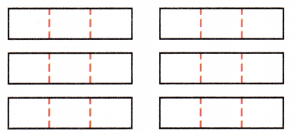To find the total number of thirds in the 6 rectangles, I can multiply the number of thirds in each rectangle by the number of rectangles.
6 ÷ $$\frac{1}{3}$$ = 6 × _________ = ___________

So, Erica has __________ one-third-size sandwich pieces.
I need to find the number of 1/3 size sandwich pieces does Eric has after she cuts 6 sandwiches into thirds.
I need to use the size of each piece of sandwich and the number of sandwiches she cuts.
Plan
I can draw a diagram to organize the information from the problem.
Then I can use the organized information to find the number of 1/3 size sandwich pieces does Eric has after she cuts 6 sandwiches into thirds.

Solve
Since Erica cuts 6 submarine sandwiches, my diagram needs to show 6 rectangles to represent the sandwiches. I can divide each of the 6 rectangles into thirds.To find the total number of thirds in the 6 rectangles, I can multiply the number of thirds in each rectangle by the number of rectangles.
6 ÷ 1/3 = 6 × 3 = 18
So, Erica has 18 one-third-size sandwich pieces.

Math Talk
Mathematical Processes

I can multiply the quotient and the divisor to see if the product is equal to the dividend.

Try Another Problem

Roberto is cutting 3 blueberry pies into halves to give to his neighbors. How many neighbors will get a $$\frac{1}{2}$$-size pie piece?
What do I need to find?

What information am I given?

Plan
What is my plan or strategy?

Solve

So, _________ neighbors will get a $$\frac{1}{2}$$-size pie piece.
I need to find how many neighbors will get 1/2 of a pie.
The information given is that Roberto is cutting 3 blueberry pies into halves to give to his neighbors. Some neighbors will get a 1/2 size pie piece.
Plan
I can draw a diagram to organize the information from the problem. Then i can use the diagram to find the number of neighbors that will get 1/2 of a pie.
Solve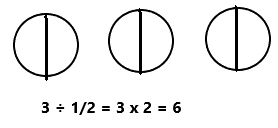So, 6 neighbors will get a 1/2 -size pie piece.

Explain how the diagram you drew for the division problem helps you write a multiplication sentence.
Since Roberto is cutting 3 pies, my diagram needs to show 3 circles to represent the pies. I can divide each of the circles into halves.
To find the total number of halves in the 3 circles, I can multiply the number of halves in each circle by the number of circles.

Share and Show

Question 1.
A chef has 5 blocks of butter. Each block weighs 1 pound. She cuts each block into fourths. How many $$\frac{1}{4}$$pound pieces of butter does the chef have?
First, draw rectangles to represent the blocks of butter.
Then, divide each rectangle into fourths.
Finally, multiply the number of fourths in each block by the number of blocks.
So, the chef has _________ one-fourth-pound pieces of butter.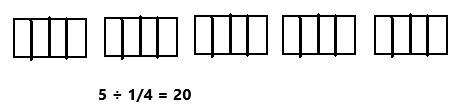So, the chef has 20 one-fourth-pound pieces of butter.
Explanation:
A chef has 5 blocks of butter. Each block weighs 1 pound. She cuts each block into fourths. First we have to draw rectangles to represent the blocks of butter as we can observe in the above image. Then, we have to divide each rectangle into fourths. Multiply the number of fourths in each block by the number of blocks. Divide 5 by 1/4 the result is 20. So, the chef has 20 one-fourth-pound pieces of butter.

Question 2.
What if the chef had 3 blocks of butter and cut the blocks into thirds? How many $$\frac{1}{3}$$-pound pieces of butter would the chef have?So, the chef has 9 one-third pound pieces of butter.
Explanation:
A chef has 3 blocks of butter. Each block weighs 1 pound. She cuts each block into thirds. First we have to draw rectangles to represent the blocks of butter as we can observe in the above image. Then, we have to divide each rectangle into thirds. Multiply the number of thirds in each block by the number of blocks. Divide 3 by 1/3 the result is 9. So, the chef has 9 one-third-pound pieces of butter.

Problem Solving

Question 3.
H.O.T. Multi-Step Julie makes a drawing that is $$\frac{1}{4}$$ the size of the original drawing. Sahil makes a drawing that is $$\frac{1}{3}$$ the size of the original. A tree in the original drawing is 12 inches tall. What will be the difference between the height of the tree in Julie and Sahil’s drawings?1/4 x 12 = 3 inches
The height of the tree in Julie’s drawing is 3 inches.
1/3 x 12 = 4 inches
The height of the tree in Sahil’s drawing is 4 inches.
4 inches – 3 inches = 1 inch
The difference between the height of the tree in Julie and Sahil’s drawings is 1 inch.
Explanation:
A tree in the original drawing is 12 inches tall. Julie makes a drawing that is 1/4 the size of the original drawing. Multiply 1/4 with 12 the product is 3. The height of the tree in Julie’s drawing is 3 inches. Sahil makes a drawing that is1/3 the size of the original. Multiply 1/3 with 12 the product is 4. The height of the tree in Sahil’s drawing is 4 inches. Subtract 3 inches from 4 inches the difference is 1 inch. The difference between the height of the tree in Julie and Sahil’s drawings is 1 inch.

Question 4.
H.O.T. Use Tools Brianna has a sheet of paper that is 6 feet long. She cuts the length of paper into sixths and then cuts the length of each of these $$\frac{1}{6}$$-pieces into thirds. How many pieces does she have? How many inches long is each piece?

Question 5.
A structure is made out of foam cubes that are each 1/4 foot tall. The height of the structure is 8 feet. How many cubes were used to build the structure?
(A) 2 cubes
(B) 16 cubes
(C) 8 cubes
(D) 32 cubes8 ÷ 1/4 = 32
To build the structure 32 cubes are used.
So, option D is correct.
Explanation:
A structure is made out of foam cubes that are each 1/4 foot tall. The height of the structure is 8 feet. Divide 8 by 1/4 the product is 32. So, 32 cubes are used to build the structure. Draw a circle for option D.

Question 6.
Use Diagrams Terrance needs to divide 9 by $$\frac{1}{2}$$. How can he find the quotient?
(A) Draw 9 rectangles and divide each in halves. Count the halves.
(B) Draw 9 rectangles and shade $$\frac{1}{2}$$ of each. Count the shaded parts.
(C) Shade a rectangle to show $$\frac{1}{2}$$, and then divide the shaded part into 9 parts. Find the amount shaded.
(D) Draw a rectangle with a length of 9 and a width of $$\frac{1}{2}$$, Find the area.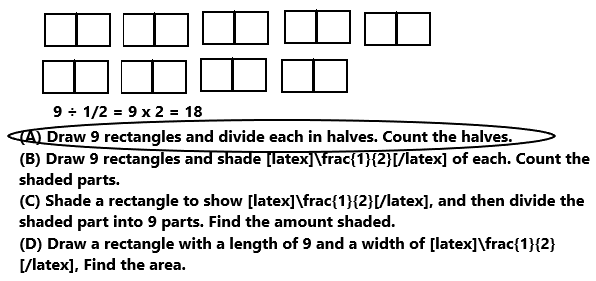Option A is correct.
Explanation:
Terrance needs to divide 9 by 1/2. First draw 9 rectangles and divide each rectangle into two halves. Then count the halves. There are 18 halves. So, draw a circle to option A.

Question 7.
Multi-Step Dorothy has a ribbon that is 3 feet long, and a ribbon that is 8 feet long. She needs to cut the ribbon into pieces that are $$\frac{1}{4}$$ foot long. How many pieces will she have in all?
(A) 12 pieces
(B) 32 pieces
(C) 11 pieces
(D) 44 pieces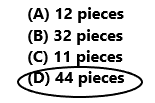3 ÷ 1/4 = 3 x 4 = 12
She have 12 pieces.
8 ÷ 1/4 = 8 x 4 = 32
12 + 32 = 44 pieces.
So, option D is correct.
Explanation:
Dorothy has a ribbon that is 3 feet long, and a ribbon that is 8 feet long. She needs to cut the ribbon into pieces that are 1/4 foot long. First divide 3 by 1/4 the result is 12 pieces. Next divide 8 by 1/4 the result is 32 pieces. Add 12 pieces with 32 pieces the sum is 44 pieces. She have 44 pieces in all. So, draw a circle for option D.

Texas Test Prep

Question 8.
Adrian made 3 carrot cakes. He cut each cake into fourths. How many $$\frac{1}{4}$$-size cake pieces does he have?
(A) 16
(B) 12
(C) 1
(D) 1$$\frac{1}{3}$$3 ÷ 1/4 = 3 x 4 = 12
He have 12 one-fourth size cake pieces.
So, option B is correct.
Explanation:
Adrian made 3 carrot cakes. He cut each cake into fourths. Divide 3 by 1/4 the result is 12. He have 12 one-fourth size cake pieces. So, draw a circle for option B.

### Texas Go Math Grade 5 Lesson 6.5 Homework and Practice Answer Key

Question 1.
Julian wants to put a border around two sides of his rectangular shaped garden. He has a 12-foot piece of lumber that will be cut into $$\frac{1}{3}$$-foot pieces. How many $$\frac{1}{3}$$-foot pieces can Julian cut from the lumber?
12 ÷ 1/3 = 12 x 3 = 36
Julian can cut 36 foot pieces from the lumber.
Explanation:
Julian wants to put a border around two sides of his rectangular shaped garden. He has a 12-foot piece of lumber that will be cut into 1/3-foot pieces. Divide 12 by 1/3 the result is 36. So, Julian can cut 36 foot pieces from the lumber.

Question 2.
The camp counselors bought a 6-pound bag of raisins for an afternoon snack for the campers. If the counselors package the raisins into $$\frac{1}{8}$$-pound individual servings, how many individual servings can they make?
6 ÷ 1/8 = 6 x 8 = 48
They can make 48 individual servings.
Explanation:
The camp counselors bought a 6-pound bag of raisins for an afternoon snack for the campers. The counselors package the raisins into 1/8-pound individual servings. Divide 6 by 1/8 the result is 48. So, they can make 48 individual servings.

Question 3.
Kim has 5 yards of denim. She cuts each yard of denim into thirds. How many $$\frac{1}{3}$$-yard pieces of denim does she have?
5 ÷ 1/3 = 5 x 3 = 15
She have 15 pieces with 1/3-yard pieces of denim.
Explanation:
Kim has 5 yards of denim. She cuts each yard of denim into thirds. Divide 5 by 1/3 the result is 15. She have 15 pieces with 1/3-yard pieces of denim.

Question 4.
If it takes Fran $$\frac{1}{5}$$ of an hour to paint one section of the fence, how many sections can she paint in 2 hours?
1/5 x 2 = 2/5
She can paint 2/5 sections in 2 hours.
Explanation:
Fran takes 1/5 of an hour to paint one section of the fence. Multiply 1/5 with 2 the product is 2/5. So, she can paint 2/5 sections in 2 hours.

Problem Solving

Question 5.
You plan to sell pumpkin pie slices at the fall festival. If you cut 4 pies into $$\frac{1}{8}$$-size pieces, how many slices will you have to sell? Describe your strategy for solving the problem.
4 ÷ 1/8 = 4 x 8 = 32
I have to sell 32 slices.
Explanation:
I plan to sell pumpkin pie slices at the fall festival. If I cut 4 pies into 1/8-size pieces. Divide 4 by 1/8 the result is 32. So, I have to sell 32 slices.

Question 6.
Marcel has 6 strips of paper. Each strip is 1 foot long. He folds each strip into $$\frac{1}{4}$$-foot sections. Describe how you can draw a diagram to find the number of sections Marcel made when he folded the paper strips.

Lesson Check

Question 7.
The students in Mrs. Lester’s fifth-grade class are planting seeds in individual pots. Mrs. Lester supplied 8 pounds of potting soil. If each pot holds $$\frac{1}{4}$$ pound of soil, how many pots can the students fill?
(A) 12
(B) 32
(C) 16
(D) 368 ÷ 1/4 = 8 x 4 = 32
The student can fill 32 pots.
So, option B is correct.
Explanation:
The students in Mrs. Lester’s fifth-grade class are planting seeds in individual pots. Mrs. Lester supplied 8 pounds of potting soil. Each pot holds 1/4 pound of soil. Divide 8 by 1/4 the result is 32. The student can fill 32 pots. So draw a circle for option B.

Question 8.
Jake’s Cafe receives a 10-pound delivery of ground beef each day. How many $$\frac{1}{4}$$-pound hamburgers can Jake make each day?
(A) 40
(B) 14
(C) 44
(D) 2010 ÷ 1/4 = 10 x 4 = 40
Jake makes 40 hamburgers each day.
So, option A is correct.
Explanation:
Jake’s Cafe receives a 10-pound delivery of ground beef each day. Divide 10 by 1/4 the result is 40. Jake makes 40 hamburgers each day. So draw a circle for option A.

Question 9.
Mrs. Miller is cutting 2 pizzas into eighths for students. How many students will get a $$\frac{1}{8}$$-size slice of pizza?
(A) 7
(B) 8
(C) 16
(D) 62 ÷ 1/8 = 2 x 8 = 16
16 students got 1/8 size slice of pizza.
So, option C is correct.
Explanation:
Mrs. Miller is cutting 2 pizzas into eighths for students. Divide 2 by 1/8 the result is 16. So, 16 students got 1/8 size slice of pizza. Draw a circle for option C.

Question 10.
A home building company purchases 24 acres of land and divides it into $$\frac{1}{2}$$-acre plots for sale. How many $$\frac{1}{2}$$-acre plots will be for sale?
(A) 6
(B) 24
(C) 12
(D) 4824 ÷ 1/2 = 24 x 2 = 48
48 plots with 1/2 acre are sale.
So, option D is correct.
Explanation:
A home building company purchases 24 acres of land and divides it into 1/2-acre plots for sale. Divide 24 by 1/2 the result is 48. So, 48 plots with 1/2 acre are sale. Draw a circle for option D.

Question 11.
Multi-Step Cleo bought 10 feet of ribbon for costumes. He used 2 feet of ribbon last week. He cut the remaining ribbon into $$\frac{1}{6}$$-foot pieces. How many pieces of ribbon does Cleo have?
(A) 48
(B) 60
(C) 72
(D) 1210 – 2 = 8
8 ÷ 1/6 = 8 x 6 = 48
Cleo have 48 pieces of ribbon.
So, option A is correct.
Explanation:
Cleo bought 10 feet of ribbon for costumes. He used 2 feet of ribbon last week. Subtract  2 feet from 10 feet the difference is 8 feet. He cut the remaining 8 feet ribbon into 1/6-foot pieces. Divide 8 by 1/6 the result is 48. So draw a circle for option A.

Question 12.
Multi-Step Reese brought 2 sandwiches for her school lunch. Each sandwich was cut into fourths. After she ate lunch, Reese has 3 pieces of sandwich left. How many pieces did Reese eat?
(A) 5
(B) 3
(C) 2
(D) 1